# Python Training Problems¶

Before diving to deep neural networks in Python, we need first to get trained with the Python programming language, at least at a decent level which will be sufficient for deep leaning with Python.

Following are a group of exercises which I have used in several courses in the past few years. As our main objective is deep learning, most of these problems are conected to data programming. They require some effort and cover the basic constructs of the language (only basic Python code is allowed - do not use any advanced Python modules yet!). Make sure you put the required effort to solve them before you proceed to Numpy and Scipy (chapter 2 and 3 just before deep learning starts).

## Problem 1: Integral Calculus¶

Write a function integrate(f, a, b, n=1000) which accepts

• A real function f(x)
• An interval points a and b
• An optional division number n (defaults to 1000)

It should compute the definite integral of f(x) over the interval (a,b) as an Riemann sum approximation. Try to test it for the following two functions:

• $f_1(x) = x^3$
• $f_2(x) = x^3\sin(x)$

Reminder from integral calculus: the Riemann Sum of a function $f(x)$ over an interval $[a,b]$ is defined by $$\int_{a}^{b} f(x)\,dx \simeq \sum_{i=0}^{n} f(x_i) \cdot (\frac{b-a}{n})$$ where $x_i$ can be chosen as $x_i = a + i \cdot \frac{(b-a)}{n}$

## Problem 2: Lists¶

Run this list comprehension in your prompt:

In :
List1 = [x**2 for x in range(5,12)]
List2 = [x+y for x in [10,20,30,40] for y in [1,2,3,4]]
List3 = [x+y for x in range(10) for y in range(10) if 2*x<y]

[1, 2, 3, 4, 5, 6, 7, 8, 9, 4, 5, 6, 7, 8, 9, 10, 7, 8, 9, 10, 11, 10, 11, 12, 13]


Figure out what is going on here, and write a nested for loop that gives you the same result. Make sure these statements make sense to you!

## Problem 3: Word Counting¶

1. Write a function word_count(file) that computes the
• number of lines
• number of words
• number of characters
in a given file. Test it on the above file. An English word should consist of English letters only (a-z, A-Z). You can ignore any other text strings.
2. Write a function word_frequency(file) which counts how many times each word appears in that book.
Hint 1: you should build a dictionary
Hint 2: Use Python's string.punctuation to remove punctuation characters from words.
3. Test your program by running it on the oliver_twist.txt book (you should get 12733 words!) Try to sort the words by frequency (from most frequent to least frequent).
4. What is the most frequent 3 letters word in this book? How many times it appears in this book?
5. How many words occur more than 1000 times?

## Problem 4: Poker¶

Each line in the file consists of 10 integers (separated by commas) which describe a Poker hand of 5 cards. Every card is represented by two integers: suit and rank. Ranks are specified by an integer 1-13: Ace=1, 2, 3, 4, 5, 6, 7, 8, 9, 10 , Jack=11, Queen=12, King=13 Suits are specified by integers 1-4: Hearts=1, Spades=2, Diamonds=3, Clubs=4

 1 heart ♥ 2 spade ♠ 3 diamond ♦ 4 club ♣

For example, the line:
1, 13, 3, 12, 2, 12, 1, 9, 4, 12
Describes the following Poker hand: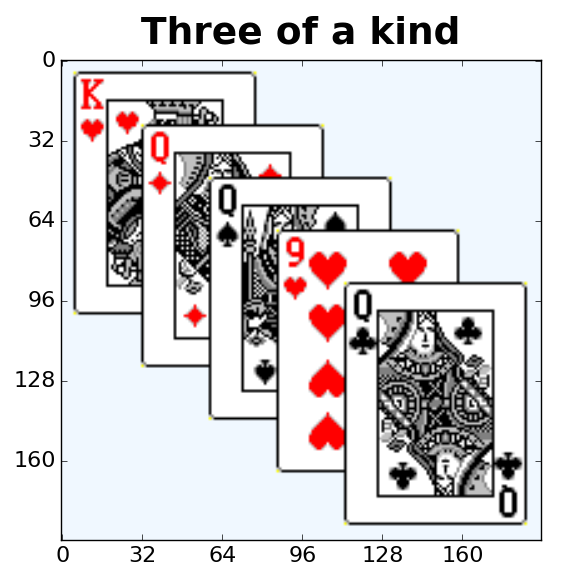This is better understood from the following table:

 1 13 3 12 2 12 1 9 4 12 ♥ K ♦ Q ♠ Q ♥ 9 ♣ Q
1. Parse the file "poker.csv" and find all Poker hands with one pair of equal ranks within five cards. Write all these hands in a new file: "pairs.csv"
2. Count how many hands with two pairs are in the file "poker.csv" ?
3. How many Straight flush hand pokers (five cards, sequentially ranked with no gaps with the same suit) are in the file?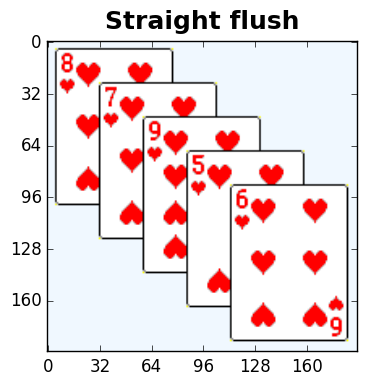## Problem 5: data processing¶

This file contains time/temperature data of 997 samples by a thermostat in a sensor unit in one day:

    00:00:32  27.12
00:01:07  33.51
00:02:29  29.95
00:04:40  18.04
00:05:29  26.30
00:07:14  17.50
...

Write Python code for answering the following questions:

1. What are the minimal and maximal temperatures ?
2. In what times the minimal temperature was obtained?
3. In what times the maximal temperature was obtained?
4. What is the average temperature?

## Problem 6: Population Data Analysis¶

This files contains fake population data (it is illegal to use real population data) of USA citizens. Each line consists of 27 data items on a person (separated by commas). It can be loaded by Microsoft Excel and looks like: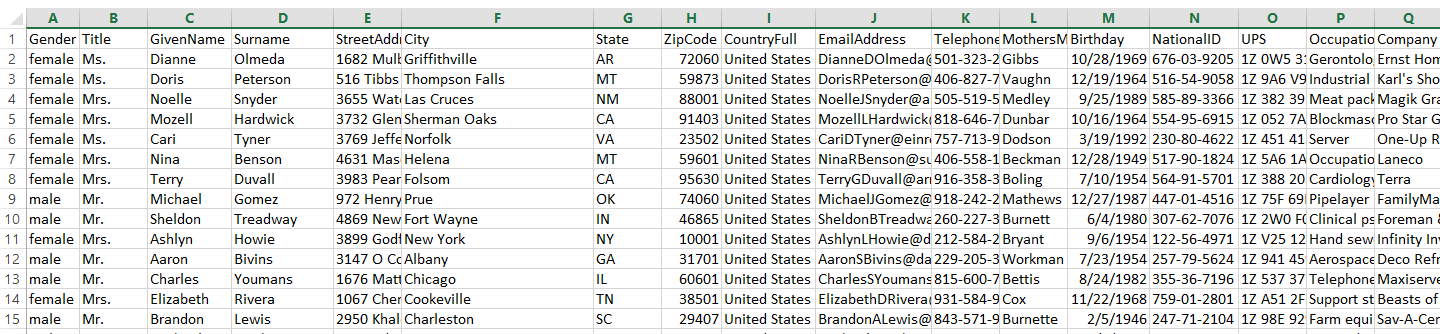Write a Python function db_query(file) for finding all the persons that meet the following criteria:

• They are from Florida or California
• Have blood type B+ or O+
• Own a Mazda car
• Were born before 1982

How many such people did you find? Write their records in a separate csv file: people.csv

## Problem 7: File Decryption¶

• http://www.samyzaf.com/ML/01-python/data/jude.txt
• http://www.samyzaf.com/ML/01-python/data/oliver_twist.txt

• Write a Python function letter_frequency(file) for counting English letters frequency in a text file. Your program output should look like this: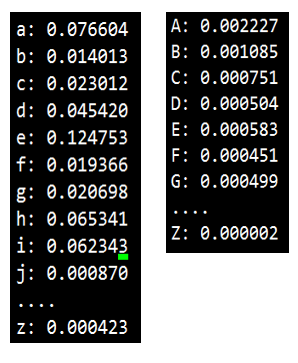• The frequency of a letter is defined as the ratio between the number of its occurrences and the total number of letters in the text (make sure to ignore characters that are not English letters!).
• Print the frequency tables for the two books.
• Do you notice any similarities between the two tables? Hint: Import the string module and look at string.letters field of the string module. Use a dictionary to hold a mapping between a letter and its number of occurrences.
• Explain what exactly happens in the following code?
In [ ]:
def random_cipher():
Letters = list(string.letters)
random.shuffle(Letters)
cipher = dict()
for letter in string.letters:
cipher[letter] = Letters.pop()
return cipher


6. Use a cipher object for encrypting a simple text file. Here is a start of your Python function:

In [ ]:
def file_encrypt(file, outfile, cipher):
letters = string.letters
f1 = open(file, 'r')
f2 = open(outfile, 'w')
...


The file_encrypt function takes a source file, a target file, and a cipher dictionary. It translates each letter in the source file to its corresponding cipher[letter].

This file was generated by applying the function file_encrypt on a very famous English book by a secret cipher object (as above). Can you use the ideas above in order to decrypt this book and its secret cipher?
Hints: Start with a utility: find_closest(x, list_of_floats) which finds the closest value in list_of_floats to x.

## Problem 8: Textfile Class¶

• This file implements the Textfile class for analyzing words frequency in large text files
• Read the usage description at the beginning of the file
• Use the Textfile class to find the 10 most used words in the book: http://www.samyzaf.com/ML/01-python/data/jude.txt
• Also indicate how many times each of these words appear in the book?
• Make sure to write a function that can be reused for other books . . .

## Problem 9: Word Frequency¶

Write a function most_common_words(file, n)
which accepts a text file name and an integer n and prints the n most frequent words in the file and their frequency count:

In [ ]:
file = "C:/DL/PYTHON/projects/proj1.txt"
most_frequent_words(file, 10)

# Output should look like this:
#    1.  the     88
#    2.  of      57
#    3.  a       52
#    4.  in      47
#    5.  is      34
#    6.  and     32
#    7.  are     27
#    8.  to      25
#    9.  numbers 16
#   10.  cards   15



## Problem 10: Advanced Object Oriented Programming¶

This is the advanced section. It might be too hard and/or too early for some students, so it can be skipped and revisited at a later stage of the course. However, keep in mind that object oriented thinking is an extremely important skill which is needed for understanding and working with deep neural networks. Therefore an investment in this project will pay off in the long run.

Start by downloding the following two files from https://github.com/samyzaf/xcanvas:

1. graphics.py
2. xcanvas.py

Add them to your work directory and check that test1 in graphics.py works for you. Try to read this file and get acquainted with the code there. The file xcanvas.py is harder to read and is not required to look at it at all. You now have a small module that enables you to draw circles, lines, and texts in all kind of sizes and colors. You should get the idea quickly by looking at the function test1 in the file graphics.py and you can learn more options and flags from: http://effbot.org/tkinterbook/canvas.htm

### Mission¶

Your mission is to write object oriented Python code for drawing neural network diagrms like this one: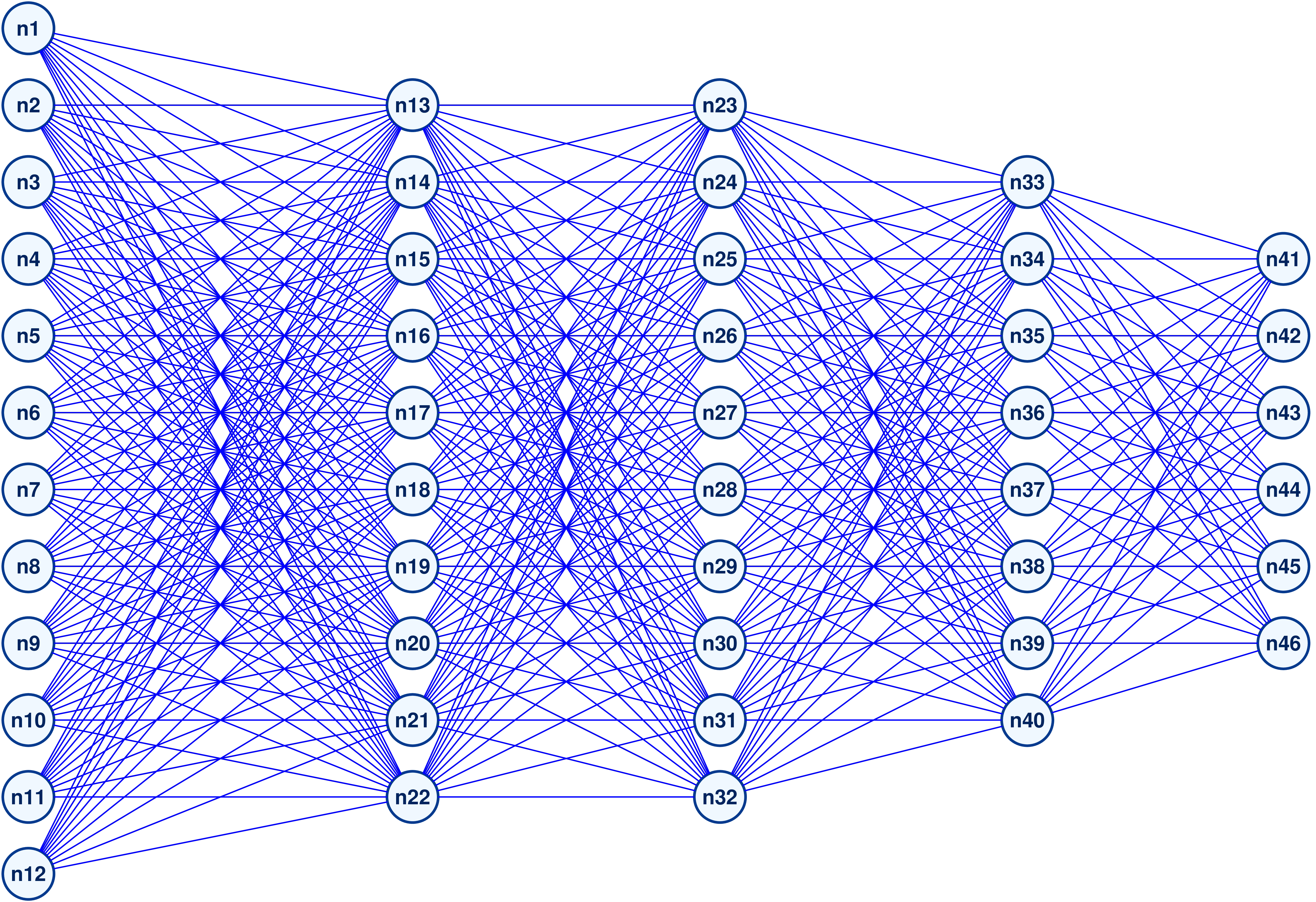To get you focused, here is a more concrete specification on how your code should look like. You will have to define 4 classes:

1. Neuron
2. Line
3. Layer
4. NeuralNetwork

Here is a more specific skeleton that we suggest you start with.

In [ ]:
from graphics import *

class Neuron():
def __init__(self, x, y, **opt):
# x, y point coordinates on the canvas
# opt is the standard Python keyword arguments dictionary
# you should set here the default values of the neuron radius,
# line width, fill color, etc.
pass

def draw(self):
# draw the neuron
pass

def bbox(self):
# neuron bounding box (to be drawn by the create_oval canvas method)
pass

class Line():
def __init__(self, n1, n2, **opt):
# n1, and n2 are two neurons
pass

def draw(self):
# draw the line connecting two neurons
pass

class Layer():
def __init__(self, name, n_neurons, **opt):
# A layer of neurons should be initialized by a name of the layer (like "layer2"),
# number of neurons, and several optional arguments such distances list, neuron labels, etc.
pass

def draw(self, ox=0, oy=0):
pass

class NeuralNetwork():
def __init__(self, name, layers, dist=100):
# A neural network object should be initialized by the network name,
# a list of layers, and a list of distances between the layers
pass

def draw(self, ox=0, oy=0):
# draw the neural network at the origin point (ox,oy)
# The origin is the left upper point on the network diagram
pass


After writing your code (which is not trivial but worth the effort!), you will be able to draw nice looking neural networks diagrams very quickly! For example, the following code

In [ ]:
lay1 = Layer("layer1", n_neurons=5, label='auto')
lay2 = Layer("layer2", n_neurons=4, label='auto')
lay3 = Layer("layer3", n_neurons=2, label='auto')
nn = NeuralNetwork("NN1", layers=[lay1, lay2, lay3], dist=[120, 90])
nn.draw(ox=50, oy=50)


Will produce the following diagram: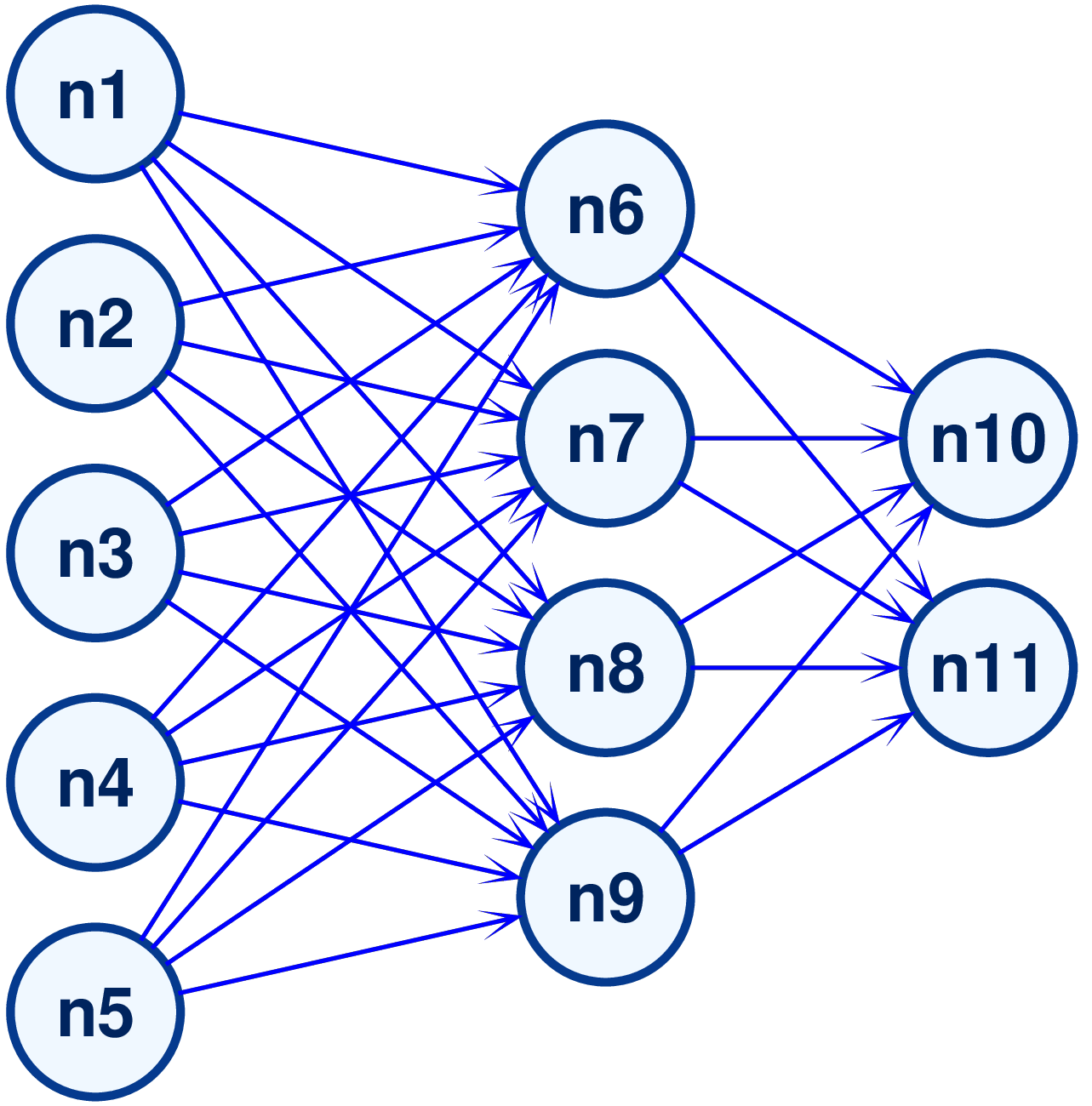Note the label flag for assigning a label to a Neural cell. The keywork 'auto' designates an automatic name based on a global counter.

The code for drawing the first diagram, should look like:

In [ ]:
l1 = Layer("layer1", 12, label='auto')
l2 = Layer("layer2", 10, label='auto')
l3 = Layer("layer3", 10, label='auto')
l4 = Layer("layer4", 8, label='auto')
l5 = Layer("layer5", 6, label='auto')
layers = [l1, l2, l3, l4, l5]
dist = [300, 240, 240, 200]
nn2 = NeuralNetwork("Network2", layers, dist)
nn2.draw(ox=50, oy=50)
show_canvas()

In :
# Fancy Notebook CSS Style
from nbstyle import *
HTML('<style>%s</style>' % (fancy(),))

Out:
In [ ]: